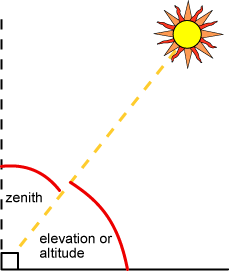# Elevation Angle

The elevation angle (used interchangeably with altitude angle) is the angular height of the sun in the sky measured from the horizontal. Confusingly, both altitude and elevation are also used to describe the height in meters above sea level. The elevation is 0° at sunrise and 90° when the sun is directly overhead (which occurs for example at the equator on the spring and fall equinoxes).

The elevation angle varies throughout the day. It also depends on the latitude of a particular location and the day of the year.An important parameter in the design of photovoltaic systems is the maximum elevation angle, that is, the maximum height of the sun in the sky at a particular time of year. This maximum elevation angle occurs at solar noon and depends on the latitude and declination angle as shown in the figure below.The maximum elevation angle at solar noon (α) is a function of latitude and the declination angle (δ).

From the previous figure, a formula for the elevation angle at solar noon can be determined according to the formula:

$\alpha =90+\phi -\delta$

When the equation above gives a number greater than 90° then subtract the result from 180°. It means the sun at solar noon is coming from the south as is typical the northern hemisphere.

where:
φ is the latitude of the location of interest (+ve for the northern hemisphere and -ve for the southern hemisphere).
δ is the declination angle, which depends on the day of the year.

At the Tropic of Cancer on the summer solstice, the sun is directly overhead and the elevation angle is 90°. In summer at latitudes between the equator and the Tropic of Cancer, the elevation angle at solar noon is greater than 90°, implying that the sunlight is coming from the north rather than from the south as in most of the northern hemisphere. Similarly, at latitudes between the equator and the Tropic of Capricorn, during some periods of the year, sunlight is incident from the south, rather than from the north.

While the maximum elevation angle is used even in very simple PV system design, more accurate PV system simulation requires the knowledge of how the elevation angle varies throughout the day. These equations are given in the following page.

The elevation, $$\alpha$$, can be found using the following formula:

## Elevation Angle

$\alpha ={\mathrm{sin}}^{-1}\left[\mathrm{sin}\delta \mathrm{sin}\phi +\mathrm{cos}\delta \mathrm{cos}\phi \mathrm{cos}\left(HRA\right)\right]$

### Zenith Angle

The zenith angle is the angle between the sun and the vertical. The zenith angle is similar to the elevation angle but it is measured from the vertical rather than from the horizontal, thus making the zenith angle = 90° - elevation.

## Zenith Angle

$\zeta ={90}^{0}-\alpha$### Sunrise and Sunset

To calculate the sunrise and sunset time the elevation is set to zero and the elevation equation above is rearranged to give:

## Sunrise Time

$Sunrise=12-\frac{1}{{15}^{0}}{\mathrm{cos}}^{-1}\left(\frac{-\mathrm{sin}\phi \mathrm{sin}\delta }{\mathrm{cos}\phi \mathrm{cos}\delta }\right)-\frac{TC}{60}$

and sunset:

## Sunset Time

$Sunset=12+\frac{1}{{15}^{0}}{\mathrm{cos}}^{-1}\left(\frac{-\mathrm{sin}\phi \mathrm{sin}\delta }{\mathrm{cos}\phi \mathrm{cos}\delta }\right)-\frac{TC}{60}$

these equations can be simplified as:

## Sunrise Time (Simplified)

$Sunrise=12-\frac{1}{{15}^{0}}{\mathrm{cos}}^{-1}\left(-\mathrm{tan}\phi \mathrm{tan}\delta \right)-\frac{TC}{60}$

## Sunset Time (simplified)

$Sunset=12+\frac{1}{{15}^{0}}{\mathrm{cos}}^{-1}\left(-\mathrm{tan}\phi \mathrm{tan}\delta \right)-\frac{TC}{60}$

where TC is the time correction.